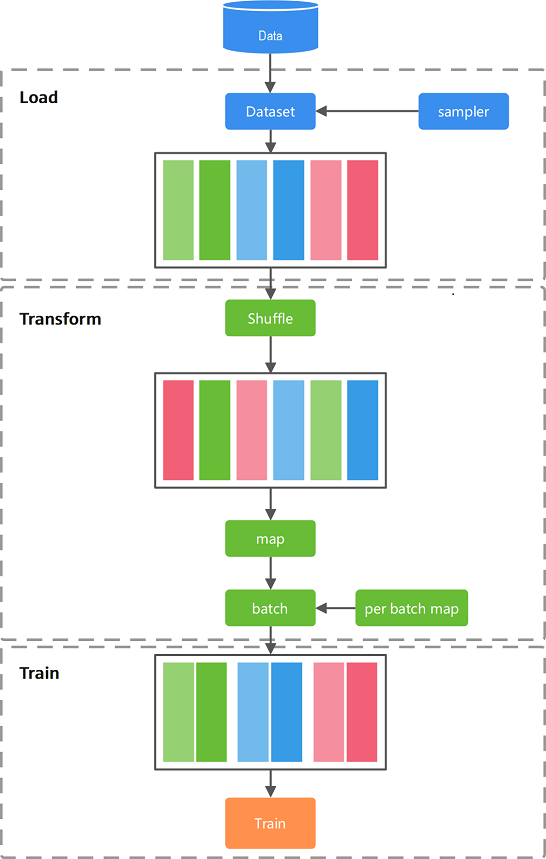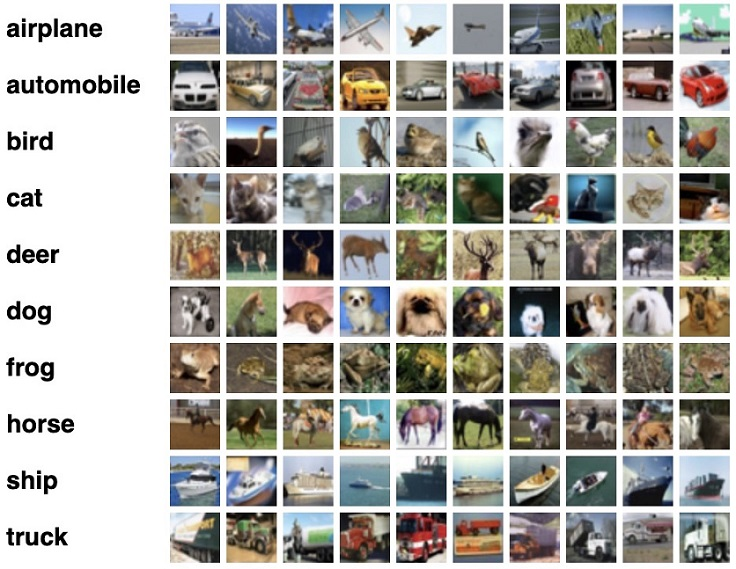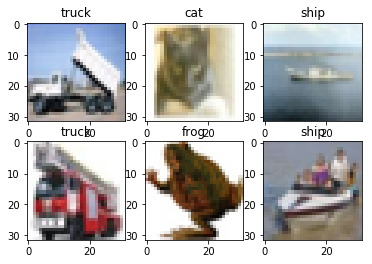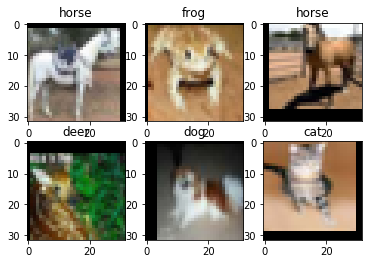# 数据处理¶

mindspore.dataset提供了部分常用数据集和标准格式数据集的加载接口，使得用户能够快速进行数据处理操作。对于图像数据集，用户可使用mindvision.dataset来加载和处理数据集。本章首先介绍如何使用mindvision.dataset.Cifar10接口加载并处理CIFAR-10数据集，然后介绍如何使用mindspore.dataset.GeneratorDataset实现自定义数据集加载。

mindvision.dataset是在mindspore.dataset的基础上开发的数据集接口。除提供数据集加载功能外，还提供数据集下载功能、数据处理和数据增强功能。

## 数据流程¶### 数据集¶

Dataset支持多种格式数据集，包括MindSpore自研数据格式MindRecord，常用的公开图像数据集和文本数据集，用户自定义的数据集等。

### 数据集加载¶

Dataset提供了多种用途的采样器（Sampler），采样器负责生成读取的index序列，Dataset负责根据index读取相应数据，帮助用户对数据集进行不同形式的采样，以满足训练需求，解决诸如数据集过大或样本类别分布不均等问题。

### 数据处理¶

Dataset将数据加载到内存后，数据按Tensor形式进行组织。同时Tensor也是数据增强操作中的基本数据结构。

## 加载数据集¶• path：数据集根目录位置。

• split：训练、测试或推理数据集，可选traintestinfer，默认为train

• download：是否下载数据集，设置True时，若数据集不存在可下载并解压数据集，默认为False

:

from mindvision.dataset import Cifar10

# 数据集根目录
data_dir = "./datasets"

# 下载解压并加载CIFAR-10训练数据集
dataset = dataset.run()


CIFAR-10数据集文件的目录结构如下：

datasets/
├── cifar-10-batches-py
│   ├── batches.meta
│   ├── data_batch_1
│   ├── data_batch_2
│   ├── data_batch_3
│   ├── data_batch_4
│   ├── data_batch_5
│   └── test_batch
└── cifar-10-python.tar.gz


## 迭代数据集¶

:

data = next(dataset.create_dict_iterator())
print(f"Data type:{type(data['image'])}\nImage shape: {data['image'].shape}, Label: {data['label']}")

data = next(dataset.create_dict_iterator(output_numpy=True))
print(f"Data type:{type(data['image'])}\nImage shape: {data['image'].shape}, Label: {data['label']}")

Data type:<class 'mindspore.common.tensor.Tensor'>
Image shape: (6, 3, 32, 32), Label: [7 1 2 8 7 8]
Data type:<class 'numpy.ndarray'>
Image shape: (6, 3, 32, 32), Label: [8 0 0 2 6 1]


## 数据处理及增强¶

### 数据处理¶

mindvision.dataset.Cifar10接口提供数据处理功能，只要设置相应的属性即可对数据进行处理操作。

• shuffle：是否打乱数据集的顺序，设置为True时打乱数据集的顺序，默认为False

• batch_size：每组包含的数据个数，batch_size=2设置每组包含2个数据， batch_size值默认大小为32。

• repeat_num：重复数据集的个数，repeat_num=1即一份数据集，repeat_num值默认为1 。

:

import numpy as np
import matplotlib.pyplot as plt

import mindspore.dataset.vision as vision

trans = [vision.HWC2CHW()] # 输入图像的shape从<H,W,C>转换成<C,H,W>
dataset = Cifar10(data_dir, batch_size=6, resize=32, repeat_num=1, shuffle=True, transform=trans)
data = dataset.run()
data = next(data.create_dict_iterator())

images = data["image"].asnumpy()
labels = data["label"].asnumpy()
print(f"Image shape: {images.shape}, Label: {labels}")

plt.figure()
for i in range(1, 7):
plt.subplot(2, 3, i)
image_trans = np.transpose(images[i-1], (1, 2, 0))
plt.title(f"{dataset.index2label[labels[i-1]]}")
plt.imshow(image_trans, interpolation="None")
plt.show()

Image shape: (6, 3, 32, 32), Label: [9 3 8 9 6 8]### 数据增强¶

• transform：对数据集图像数据进行增强。

• target_transform：对数据集标签数据进行处理。

:

import numpy as np
import matplotlib.pyplot as plt

import mindspore.dataset.vision as vision

# 图像增强
trans = [
vision.RandomCrop((32, 32), (4, 4, 4, 4)), # 对图像进行自动裁剪
vision.RandomHorizontalFlip(prob=0.5), # 对图像进行随机水平翻转
vision.HWC2CHW(), # (h, w, c)转换为(c, h, w)
]

dataset = Cifar10(data_dir, batch_size=6, resize=32, transform=trans)
data = dataset.run()
data = next(data.create_dict_iterator())
images = data["image"].asnumpy()
labels = data["label"].asnumpy()
print(f"Image shape: {images.shape}, Label: {labels}")

plt.figure()
for i in range(1, 7):
plt.subplot(2, 3, i)
image_trans = np.transpose(images[i-1], (1, 2, 0))
plt.title(f"{dataset.index2label[labels[i-1]]}")
plt.imshow(image_trans, interpolation="None")
plt.show()

Image shape: (6, 3, 32, 32), Label: [7 6 7 4 5 3]